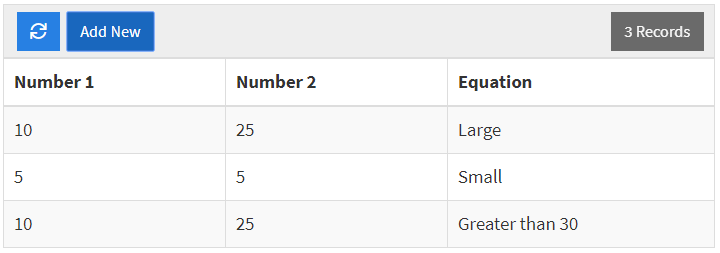This article provides details on our Other Functions that are available for use with the Equation Field.The IF function generates a result based on the given condition. This function expects 3 fields as input. In the image below, the value (a) represents the condition where (b) and (c) represent the possible results depending on whether the condition in met.1. The condition portion of this function is an input value that can vary depending on your needs. For our example, this value is set as ({Number 1}+{Number 2})>30 so the sum of the two fields {Number 1} and {Number 2} must be greater than 30 for the condition to be met. Within this portion of the function, you can perform math operations (addition, subtraction, multiplication, etc.) and you may use a number of comparison operators. Here is a list of common comparison operators you may use with this function:
• a>b (a is greater than b)
• a<b (a is less than b)
• a>=b (a is greater than or equal to b)
• a<=b (a is less than or equal to b)
• a=b (a equals b)
• a!=b (a does not equal b)
2. If the condition is true, then the function will set the value of the field to (b). This value can be a field (as shown in this example) or it can be a static value that is defined within the function.
3. If the condition is false, then the function will set the value fo the field to (c). This value can be defined as a field or static value similar to (b).

Below are some result values for the example we have used. Since (b) is a field value, we allow th flexibility to define the value for each record.